K. G Sreekumar

•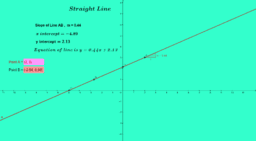Equation of straight line with two given points

Activity

K. G Sreekumar

•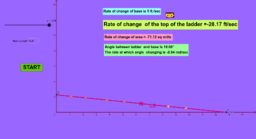Activity

K. G Sreekumar

•Concavity of graphs of functions - Concave up and down

Activity

K. G Sreekumar

•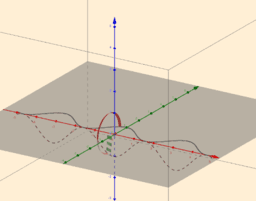Volume of Solids by revolution (nct1)

Activity

K. G Sreekumar

•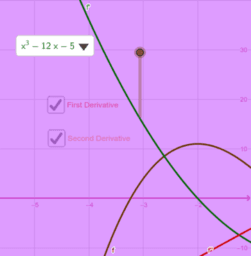Relation between function and its derivative(Nct2)

Activity

K. G Sreekumar

•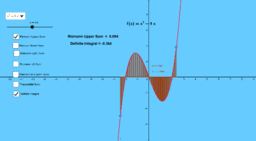Comparison between different Riemann Integrals

Activity

K. G Sreekumar

•Area between lines and curves

Activity

K. G Sreekumar

•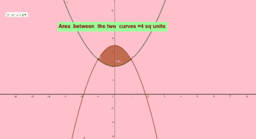Area between parabola and a curve

Activity

K. G Sreekumar

•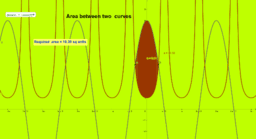Area between two curves

Activity

K. G Sreekumar

•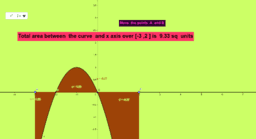Area under a curve

Activity

K. G Sreekumar

•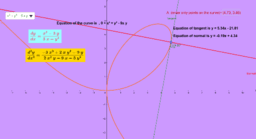Derivative of implicitly defined functions

Activity

K. G Sreekumar

•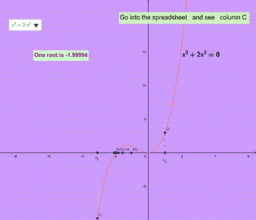Bisection method to find a real root of an equation

Activity

K. G Sreekumar

•Newton Raphson method

Activity

K. G Sreekumar

•Orthogonal trajectories self

Activity

K. G Sreekumar

•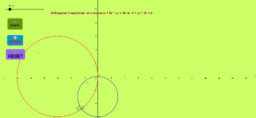Orthogonal trajectories 1

Activity

K. G Sreekumar

•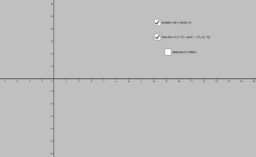Solution of ODE and integration - Examples

Activity

K. G Sreekumar

•Zeros of Polynomials- Example

Activity

K. G Sreekumar

•Vertical line test

Activity

K. G Sreekumar

•Determine Which of the following equations define functions

Activity

K. G Sreekumar

•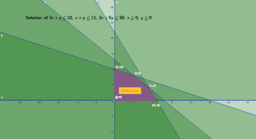Solution of Linear inequalities in two variables

Activity

K. G Sreekumar

•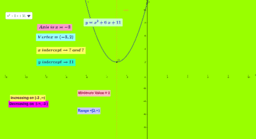Activity

K. G Sreekumar

•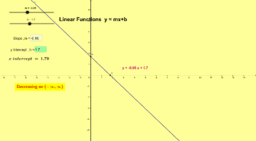Linear functions -Examples

Activity

K. G Sreekumar

•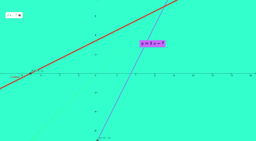Inverse of functions -Examples

Activity

K. G Sreekumar

•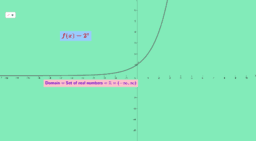Exponential and logarithmic functions

Activity

K. G Sreekumar